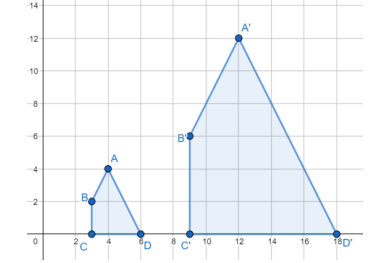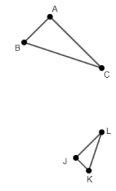# MA.912.GR.2.8Export Print
Apply an appropriate transformation to map one figure onto another to justify that the two figures are similar.

### Clarifications

Clarification 1: Instruction includes showing that the corresponding sides are proportional, and the corresponding angles are congruent.
General Information
Subject Area: Mathematics (B.E.S.T.)
Strand: Geometric Reasoning
Status: State Board Approved

## Benchmark Instructional Guide

### Terms from the K-12 Glossary

• Coordinate Plane
• Dilation
• Origin
• Reflection
• Rigid Transformation
• Rotation
• Scale Factor
• Translation

### Vertical Alignment

Previous Benchmarks

Next Benchmarks

### Purpose and Instructional Strategies

In grade 8, students identify the scale factor of a dilation given the preimage and the image and learn that when the transformation is a dilation, this transformation does not preserve congruence. The preimage is mapped onto a scaled copy of itself. In Geometry, students determine whether two figures are similar and justify their answers using a dilation (non-rigid motion) or a sequence that includes at least one dilation. This leads to the definition of similarity in terms of dilations and rigid transformations. (MTR.5.1)
• When identifying the dilation, specify the center and scale factor of the dilation.
• Instruction includes describing the transformation using words and using coordinates.
• It is important to identify corresponding parts between the preimage and the image leading to the similarity statement and the congruence of the corresponding angles and the proportionality of the corresponding sides.
• Instruction includes using examples to compare transformations. Include situations where the preimage and the image are not similar to show how dilations will fail mapping one figure onto the other. (MTR.4.1)
• When proving two triangles are similar, it is important to discuss with the students the effects of choosing which one of the triangles is the pre-image. This affects the scale factor of the dilation.
• For example, when proving that ΔABC and ΔPQR are similar, the scale factor of the dilation that maps ΔABC onto ΔPQR is $k$, while the scale factor of the dilation that maps ΔPQR onto ΔABC is $\frac{\text{1}}{\text{k}}$.

### Common Misconceptions or Errors

• When determining the scale factor of a dilation, students may misidentify the preimage and image, leading to an incorrect scale factor.

• A dilation with scale factor 3 was used to map polygon ABCD onto polygon A'B'C'D'• Part A. Fill in the blanks with either congruent or proportional.
If the figures are similar, the corresponding sides are _______ and corresponding angles are________.
• Part B. Identify the sequence of rigid and non-rigid transformations that maps polygon ABCD onto polygon A'B'C'D'
• Part B. Use the definition of similarity to prove that polygon ABCD is similar to polygon A'B'C'D'. You may need to decompose the polygon into triangles and rectangles.

### Instructional Items

Instructional Item 1
• In triangles ABD and JKL, $m$A = $m$J, $m$C = $m$L, and AC = 2JL• Part A. Describe a sequence of transformations that maps ΔABC onto ΔJKL.
• Part B. Based on the transformations chosen, determine whether ΔABC is congruent or similar to ΔJKL.

*The strategies, tasks and items included in the B1G-M are examples and should not be considered comprehensive.

## Related Courses

This benchmark is part of these courses.
1200400: Foundational Skills in Mathematics 9-12 (Specifically in versions: 2014 - 2015, 2015 - 2022, 2022 and beyond (current))
1206310: Geometry (Specifically in versions: 2014 - 2015, 2015 - 2022, 2022 and beyond (current))
1206320: Geometry Honors (Specifically in versions: 2014 - 2015, 2015 - 2022, 2022 and beyond (current))
1206315: Geometry for Credit Recovery (Specifically in versions: 2014 - 2015, 2015 - 2022, 2022 and beyond (current))
7912065: Access Geometry (Specifically in versions: 2015 - 2022, 2022 and beyond (current))

## Related Access Points

Alternate version of this benchmark for students with significant cognitive disabilities.
MA.912.GR.2.AP.8: Identify an appropriate transformation to map one figure onto another to show that the two figures are similar.

## Related Resources

Vetted resources educators can use to teach the concepts and skills in this benchmark.

## Formative Assessments

Justifying a Proof of the AA Similarity Theorem:

Students are asked to justify statements of a proof of the AA Similarity Theorem.

Type: Formative Assessment

Prove the AA Similarity Theorem:

Students will indicate a complete proof of the AA Theorem for triangle similarity.

Type: Formative Assessment

Proving Similarity:

Students are asked to explain similarity in terms of transformations.

Type: Formative Assessment

Showing Similarity:

Students are asked to use the definition of similarity in terms of similarity transformations to determine whether or not two quadrilaterals are similar.

Type: Formative Assessment

To Be or Not To Be Similar:

Students are asked to use the definition of similarity in terms of similarity transformations to determine whether or not two triangles are similar.

Type: Formative Assessment

## Perspectives Video: Professional/Enthusiast

Making Candy: Uniform Scaling:

Don't be a shrinking violet. Learn how uniform scaling is important for candy production.

Type: Perspectives Video: Professional/Enthusiast

## Text Resource

Math for Hungry Birds:

This informational text resource is intended to support reading in the content area. A new study indicates that the flying patterns of hunting albatrosses may resemble mathematical designs called fractals. This article describes the basics of fractals and why scientists think the albatross may hunt in such patterns. As it turns out, many animals may use math to find food!

Type: Text Resource

## MFAS Formative Assessments

Justifying a Proof of the AA Similarity Theorem:

Students are asked to justify statements of a proof of the AA Similarity Theorem.

Prove the AA Similarity Theorem:

Students will indicate a complete proof of the AA Theorem for triangle similarity.

Proving Similarity:

Students are asked to explain similarity in terms of transformations.

Showing Similarity:

Students are asked to use the definition of similarity in terms of similarity transformations to determine whether or not two quadrilaterals are similar.

To Be or Not To Be Similar:

Students are asked to use the definition of similarity in terms of similarity transformations to determine whether or not two triangles are similar.

## Student Resources

Vetted resources students can use to learn the concepts and skills in this benchmark.

## Perspectives Video: Professional/Enthusiast

Making Candy: Uniform Scaling:

Don't be a shrinking violet. Learn how uniform scaling is important for candy production.

Type: Perspectives Video: Professional/Enthusiast

## Parent Resources

Vetted resources caregivers can use to help students learn the concepts and skills in this benchmark.

## Perspectives Video: Professional/Enthusiast

Making Candy: Uniform Scaling:

Don't be a shrinking violet. Learn how uniform scaling is important for candy production.

Type: Perspectives Video: Professional/Enthusiast